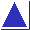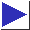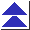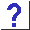# comp.lang.c FAQ list ·Question 13.6

Q: How can I split up a string into whitespace-separated fields?
How can I duplicate the process by which main() is handed argc and argv?

A: The only Standard function available for this kind of ``tokenizing'' is strtok, although it can be tricky to use [footnote] and it may not do everything you want it to. (For instance, it does not handle quoting.) Here is a usage example, which simply prints each field as it's extracted:

```#include <stdio.h>
#include <string.h>
char string[] = "this is a test";	/* not char *; see Q 16.6 */
char *p;
for(p = strtok(string, " \t\n"); p != NULL;
p = strtok(NULL, " \t\n"))
printf("\"%s\"\n", p);
```

As an alternative, here is a routine I use for building an argv all at once:

```#include <ctype.h>

int makeargv(char *string, char *argv[], int argvsize)
{
char *p = string;
int  i;
int argc = 0;

for(i = 0; i < argvsize; i++) {
while(isspace(*p))
p++;

if(*p != '\0')
argv[argc++] = p;
else {
argv[argc] = 0;
break;
}

/* scan over arg */
while(*p != '\0' && !isspace(*p))
p++;
/* terminate arg: */
if(*p != '\0' && i < argvsize-1)
*p++ = '\0';
}

return argc;
}
```

Calling makeargv is straightforward:

```	char *av;
int i, ac = makeargv(string, av, 10);
for(i = 0; i < ac; i++)
printf("\"%s\"\n", av[i]);
```

If you want each separator character to be significant, for instance if you want two tabs in a row to indicate an omitted field, it's probably more straightforward to use strchr:

```#include <stdio.h>
#include <string.h>

char string[] = "this\thas\t\tmissing\tfield";
char *p = string;

while(1) {		/* break in middle */
char *p2 = strchr(p, '\t');
if(p2 != NULL)
*p2 = '\0';
printf("\"%s\"\n", p);
if(p2 == NULL)
break;
p = p2 + 1;
}
```

All the code fragments presented here modify the input string, by inserting \0's to terminate each field (meaning that the string must be writable; see question 1.32). If you'll need the original string later, make a copy before breaking it up.

References: K&R2 Sec. B3 p. 250
ISO Sec. 7.11.5.8
H&S Sec. 13.7 pp. 333-4
PCS p. 178